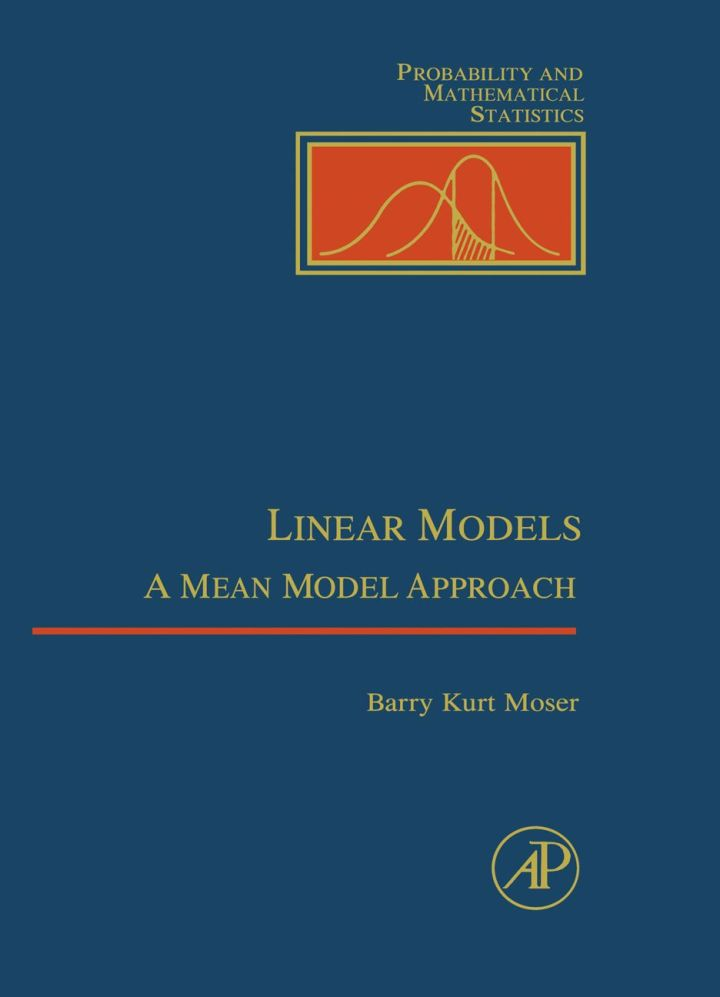# Linear Models: A Mean Model Approach

## Linear Models: A Mean Model Approach

By Moser, William R.

### Rent or Buy eTextbook

Expires on Apr 13th, 2022
\$120.95

Publisher List Price: \$139.99
Savings: \$19.04

Linear models, normally presented in a highly theoretical and mathematical style, are brought down to earth in this comprehensive textbook. Linear Models examines the subject from a mean model perspective, defining simple and easy-to-learn rules for building mean models, regression models, mean vectors, covariance matrices and sums of squares matrices for balanced and unbalanced data sets. The author includes both applied and theoretical discussions of the multivariate normal distribution, quadratic forms, maximum likelihood estimation, less than full rank models, and general mixed models. The mean model is used to bring all of these topics together in a coherent presentation of linear model theory.

Key Features
* Provides a versatile format for investigating linear model theory, using the mean model
* Uses examples that are familiar to the student:
* design of experiments, analysis of variance, regression, and normal distribution theory
* Includes a review of relevant linear algebra concepts
* Contains fully worked examples which follow the theorem/proof presentation

Subject: Mathematics & Statistics -> Calculus -> CalculusPublisher: Elsevier S & T 10/1996
Imprint: Academic Press
Language: English
Length: 228 pages

ISBN 10: 012508465X
ISBN 13: 9780125084659
Print ISBN: 9780125084659

Live Chats